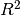# User guide: create your own scikit-learn estimator¶

## Estimator¶

The central piece of transformer, regressor, and classifier is `sklearn.base.BaseEstimator`. All estimators in scikit-learn are derived from this class. In more details, this base class enables to set and get parameters of the estimator. It can be imported as:

```>>> from sklearn.base import BaseEstimator
```

Once imported, you can create a class which inherate from this base class:

```>>> class MyOwnEstimator(BaseEstimator):
...     pass
```

## Transformer¶

Transformers are scikit-learn estimators which implement a `transform` method. The use case is the following:

• at `fit`, some parameters can be learned from `X` and `y`;

• at `transform`, X will be transformed, using the parameters learned during `fit`.

In addition, scikit-learn provides a mixin, i.e. `sklearn.base.TransformerMixin`, which implement the combination of `fit` and `transform` called `fit_transform`:

One can import the mixin class as:

```>>> from sklearn.base import TransformerMixin
```

Therefore, when creating a transformer, you need to create a class which inherits from both `sklearn.base.BaseEstimator` and `sklearn.base.TransformerMixin`. The scikit-learn API imposed `fit` to return ``self``. The reason is that it allows to pipeline `fit` and `transform` imposed by the `sklearn.base.TransformerMixin`. The `fit` method is expected to have `X` and `y` as inputs. Note that `transform` takes only `X` as input and is expected to return the transformed version of `X`:

```>>> class MyOwnTransformer(BaseEstimator, TransformerMixin):
...     def fit(self, X, y=None):
...         return self
...     def transform(self, X):
...         return X
```

We build a basic example to show that our `MyOwnTransformer` is working within a scikit-learn `pipeline`:

```>>> from sklearn.datasets import load_iris
>>> from sklearn.pipeline import make_pipeline
>>> from sklearn.linear_model import LogisticRegression
>>> pipe = make_pipeline(MyOwnTransformer(),
...                      LogisticRegression(random_state=10,
...                                         solver='lbfgs'))
>>> pipe.fit(X, y)
Pipeline(...)
>>> pipe.predict(X)
array([...])
```

## Predictor¶

### Regressor¶

Similarly, regressors are scikit-learn estimators which implement a `predict` method. The use case is the following:

• at `fit`, some parameters can be learned from `X` and `y`;

• at `predict`, predictions will be computed using `X` using the parameters learned during `fit`.

In addition, scikit-learn provides a mixin, i.e. `sklearn.base.RegressorMixin`, which implements the `score` method which computes thescore of the predictions.

One can import the mixin as:

```>>> from sklearn.base import RegressorMixin
```

Therefore, we create a regressor, `MyOwnRegressor` which inherits from both `sklearn.base.BaseEstimator` and `sklearn.base.RegressorMixin`. The method `fit` gets `X` and `y` as input and should return `self`. It should implement the `predict` function which should output the predictions of your regressor:

```>>> import numpy as np
>>> class MyOwnRegressor(BaseEstimator, RegressorMixin):
...     def fit(self, X, y):
...         return self
...     def predict(self, X):
...         return np.mean(X, axis=1)
```

We illustrate that this regressor is working within a scikit-learn pipeline:

```>>> from sklearn.datasets import load_diabetes
>>> pipe = make_pipeline(MyOwnTransformer(), MyOwnRegressor())
>>> pipe.fit(X, y)
Pipeline(...)
>>> pipe.predict(X)
array([...])
```

Since we inherit from the `sklearn.base.RegressorMixin`, we can call the `score` method which will return thescore:

```>>> pipe.score(X, y)
-3.9...
```

### Classifier¶

Similarly to regressors, classifiers implement `predict`. In addition, they output the probabilities of the prediction using the `predict_proba` method:

• at `fit`, some parameters can be learned from `X` and `y`;

• at `predict`, predictions will be computed using `X` using the parameters learned during `fit`. The output corresponds to the predicted class for each sample;

• `predict_proba` will give a 2D matrix where each column corresponds to the class and each entry will be the probability of the associated class.

In addition, scikit-learn provides a mixin, i.e. `sklearn.base.ClassifierMixin`, which implements the `score` method which computes the accuracy score of the predictions.

One can import this mixin as:

```>>> from sklearn.base import ClassifierMixin
```

Therefore, we create a classifier, `MyOwnClassifier` which inherits from both `slearn.base.BaseEstimator` and `sklearn.base.ClassifierMixin`. The method `fit` gets `X` and `y` as input and should return `self`. It should implement the `predict` function which should output the class inferred by the classifier. `predict_proba` will output some probabilities instead:

```>>> class MyOwnClassifier(BaseEstimator, ClassifierMixin):
...     def fit(self, X, y):
...         self.classes_ = np.unique(y)
...         return self
...     def predict(self, X):
...         return np.random.randint(0, self.classes_.size,
...                                  size=X.shape)
...     def predict_proba(self, X):
...         pred = np.random.rand(X.shape, self.classes_.size)
...         return pred / np.sum(pred, axis=1)[:, np.newaxis]
```

We illustrate that this regressor is working within a scikit-learn pipeline:

```>>> X, y = load_iris(return_X_y=True)
>>> pipe = make_pipeline(MyOwnTransformer(), MyOwnClassifier())
>>> pipe.fit(X, y)
Pipeline(...)
```

Then, you can call `predict` and `predict_proba`:

```>>> pipe.predict(X)
array([...])
>>> pipe.predict_proba(X)
array([...])
```

Since our classifier inherits from `sklearn.base.ClassifierMixin`, we can compute the accuracy by calling the `score` method:

```>>> pipe.score(X, y)
0...
```